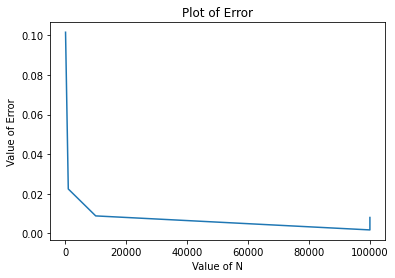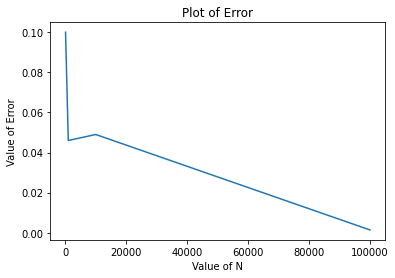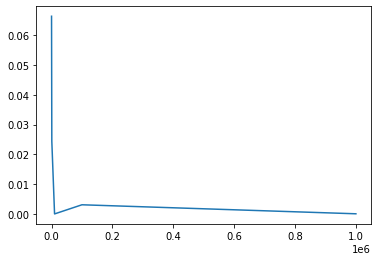# Estimation of Value of Pi From Area of Circle in Two Dimension Using Monte Carlo

Here, we have value of hypersphere in n dimension

$V_n(R) = \frac{\pi^\frac{n}{2}}{\frac{n}{2}!}R^n$

When n = 2, then above equation becomes, $$V_2(R) = \frac{\pi^\frac{2}{2}}{\frac{2}{2}!}R^2$$ $$V_2(R) = \pi R^2$$

In a 2-D plane, the goal is to mimic random (x, y) points with a domain that is a square of 2r units centered on (0,0). Consider an inscribed square-shaped circle with the same radius r inside the same domain. The ratio between the number of points inside the circle and the overall number of generated points is then determined.

In our case r = 1 radius of circle

L = 2r length of square

v = L^2 volume of square = 4

In 2D

v = 𝜋𝑅2

𝜋 = 4

import random
import math
import numpy as np
import matplotlib.pyplot as plt


N = [100,1000,10000,100000,100000]
E = []

true_value = 3.141592
for j in range(len(N)):
c= 0
s = 0
I = 0

for i in range(N[j]):
x = random.random()
y = random.random()
d = x*x + y*y
if d <= 1:
c +=1
s +=1
I += 1

pi = 4 * (c/s)
print(f'Calculated value of pi: {pi}')
error = abs(pi - true_value)
print(f'Estimated value of error: {error}')
E.append(error)


Calculated value of pi: 3.04
Estimated value of error: 0.10159200000000013
Calculated value of pi: 3.164
Estimated value of error: 0.022407999999999983
Calculated value of pi: 3.1504
Estimated value of error: 0.008807999999999705
Calculated value of pi: 3.14332
Estimated value of error: 0.0017279999999999518
Calculated value of pi: 3.1496
Estimated value of error: 0.008007999999999793


# Plot of Number VS Error

plt.plot(N,E)
plt.title("Plot of Error")
plt.xlabel("Value of N")
plt.ylabel("Value of Error")

Text(0, 0.5, 'Value of Error')# Estimation of Value of Pi From Volume of Sphere in Three Dimension

Here, we have value of hypersphere in n dimension

$V_n(R) = \frac{\pi^\frac{n}{2}}{\frac{n}{2}!}R^n$

When n = 3, then above equation becomes, $$V_3(R) = \frac{\pi^\frac{3}{2}}{\frac{3}{2}!}R^3$$ $$V_3(R) =0.752252 \pi^\frac{3}{2} R^3$$

In our case r = 1 radius of circle

L = 2r length of square

v = L^3 volume of square = 8

In 3D

v = 4/3𝜋𝑅3

8 = 4/3 𝜋

𝜋 = 6

import random
N = [100,1000,10000,100000]
E = []
true_value = 3.14
for i in range(len(N)):
I = 0
for j in range(N[i]):
x = random.random()
y = random.random()
z = random.random()

r = x**2 + y**2+ z**2
if r<1:
I = I + 1

ratio = I/N[i]
Pi = ratio * 6
print(f'Calculated value of pi: {Pi}')
error = abs(Pi - true_value)
print(f'Estimated value of error: {error}')
E.append(error)


Calculated value of pi: 3.24
Estimated value of error: 0.10000000000000009
Calculated value of pi: 3.186
Estimated value of error: 0.04599999999999982
Calculated value of pi: 3.189
Estimated value of error: 0.04899999999999993
Calculated value of pi: 3.1386000000000003
Estimated value of error: 0.0013999999999998458


# Plot of Number VS Error

plt.plot(N,E)
plt.title("Plot of Error")
plt.xlabel("Value of N")
plt.ylabel("Value of Error")

Text(0, 0.5, 'Value of Error')# Estimation of Value of Pi From Area of Circle in Four Dimension

Here, we have value of hypersphere in n dimension

$V_n(R) = \frac{\pi^\frac{n}{2}}{\frac{n}{2}!}R^n$

When n = 4, then above equation becomes, $$V_4(R) = \frac{\pi^\frac{4}{2}}{\frac{4}{2}!}R^4$$ $$V_4(R) = \frac{1}{2}(\pi R^2 )^2$$

In our case r = 1 radius of circle

L = 2r length of square

v = L^3 volume of square = 16

In 4D

v = 1/2 𝜋2𝑅4

16 = 1/2 𝜋2

𝜋 = 32^0.5

# Estimation of Value of Pi from the integration function

$$\pi = \int_{0}^1 \frac{1}{1+ x^2}dx$$ By Hand

$= [tan^-(x)]_{0}^1$ $= tan^-(1) - tan^-(0)$

$$= \pi - 0$$ $$= 3.141592653589793238$$

# Estimation of value of pi using Integration Method

Algorithm:

1. Import random

2. Import math

3. Import numpy

4. a = 0

5. b = 1

6. integral = 0

7. i = 0

8. while i < 1000

9. x = random.random()

10. integral += 1/1 + x**2

11. i = i + 1

12. ans = integral* (b-a)/float(N)

13. Print(ans)


N = [100,1000,10000,100000,1000000]
print("True Value of Pi")
true_value = 3.141592653589793238
print(true_value)
Error = []
for i in range(len(N)):
a = 0
b = 1
integral = 0
for j in range(N[i]):
x = random.random()
integral += 4 * 1/(1 + x**2)
ans = integral * (b-a)/float(N[i])
error = abs(ans - true_value)
Error.append(error)
#print(ans)
print(f'Calclated Value of Pi : {ans}')
print(f'Error Value of pi: {Error}')

True Value of Pi
3.141592653589793
Calclated Value of Pi : 3.2079305434667744
Calclated Value of Pi : 3.1171874694431705
Calclated Value of Pi : 3.141631082310233
Calclated Value of Pi : 3.138488698353392
Calclated Value of Pi : 3.14167462845529
Error Value of pi: [0.0663378898769813, 0.024405184146622627, 3.8428720440020214e-05, 0.003103955236400946, 8.197486549699207e-05]


# Plot of Number Vs Error

plt.plot(N,E)
plt.title("Plot of Error")
plt.xlabel("Value of N")
plt.ylabel("Value of Error")

[<matplotlib.lines.Line2D at 0x2054093c490>]Tags:

Categories:

Updated: